# NCERT Solutions for Class 10 Maths Exercise 14.1## myCBSEguide App

CBSE, NCERT, JEE Main, NEET-UG, NDA, Exam Papers, Question Bank, NCERT Solutions, Exemplars, Revision Notes, Free Videos, MCQ Tests & more.

NCERT solutions for Maths Statistics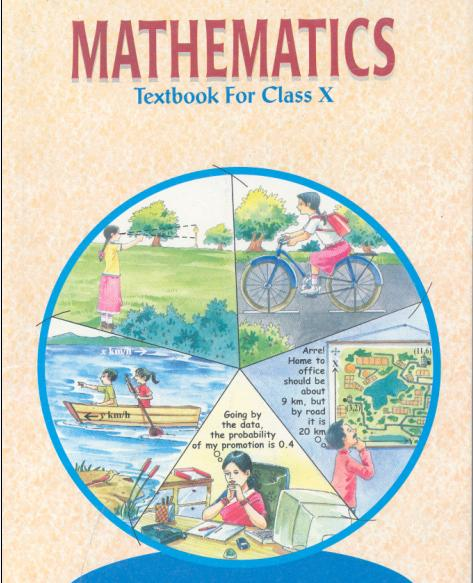## NCERT Solutions for Class 10 Maths Statistics

###### 1. A survey was conducted by a group of students as a part of their environment awareness programme, in which they collected the following data regarding the number of plants in 20 houses in a locality. Find the mean number of plants per house.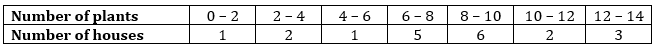Which method did you use for finding the mean and why?

Ans. Since, number of plants and houses are small in their values, so we use direct method.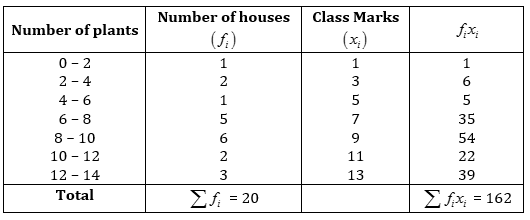Mean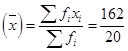= 8.1

Hence mean number of plants per house is 8.1.

NCERT Solutions for Class 10 Maths Exercise 14.1

###### 2. Consider the following distribution of daily wages of 50 workers of a factory.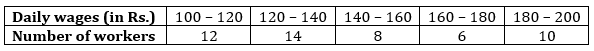Find the mean daily wages of the workers of the factory by using an appropriate method.

Ans.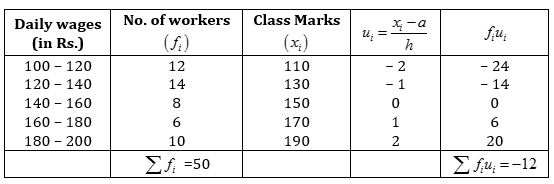From given data, Assume mean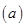= 150, Width of the class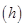= 20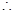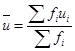=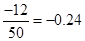Using formula, Mean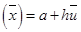=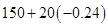= 150 – 4.8 = 145.2

Hence mean daily wages of the workers of factory is Rs. 145.20.

NCERT Solutions for Class 10 Maths Exercise 14.1

###### 3. The following distribution shows the daily pocket allowance of children of a locality. The mean pocket allowance is Rs.18. Find the missing frequency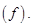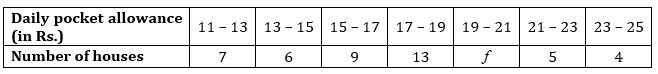Ans.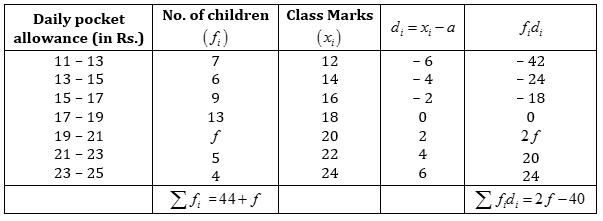From given data, Assume mean= 18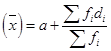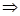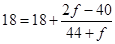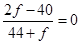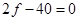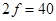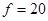Hence missing frequency is 20.

NCERT Solutions for Class 10 Maths Exercise 14.1

###### 4. Thirty women were examined in a hospital by a doctor and the number of heart beats per minute were recorded and summarized as follows: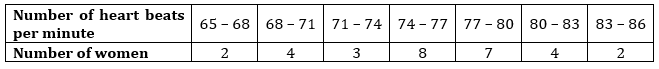Ans.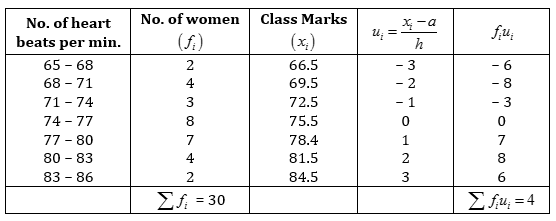From given data, Assume mean= 75.5, Width of the class= 3=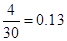(approx.)

Using formula, Mean= 75.5 + 3 (0.13) = 75.5 + 0.39 = 78.89

Hence mean heart beat per minute for women is 78.89.

NCERT Solutions for Class 10 Maths Exercise 14.1

###### 5. In a retail market, fruit vendors were selling mangoes kept in packing boxes. These boxes contained varying number mangoes. The following was the distribution of mangoes according to the number of boxes.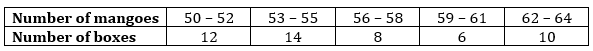Find the mean number of mangoes kept in a packing box. Which method of finding the mean did you choose?

Ans. Since value of number of mangoes and number of boxes are large numerically. So we use step-deviation method.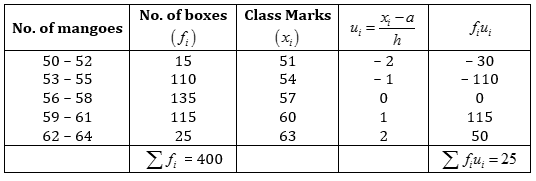From given data, Assume mean= 57, Width of the class= 3=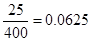(approx.)

Using formula, Mean= 57 + 3 (0.0625) = 57 + 0.1875 = 57.1875 = 57.19 (approx.)

Hence mean number of mangoes kept in a packing box is 57.19.

NCERT Solutions for Class 10 Maths Exercise 14.1

###### 6. The table below shows the daily expenditure on food of 25 households in a locality: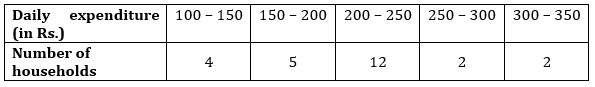Find the mean daily expenditure on food by a suitable method.

Ans.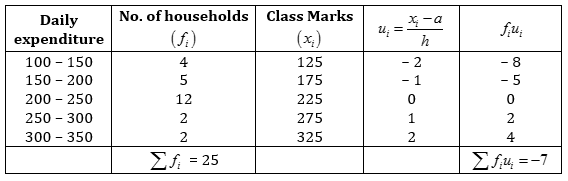From given data, Assume mean= 225, Width of the class= 50=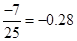Using formula, Mean= 225 + 50 (– 0.28) = 225 – 14 = 211

Hence mean daily expenditure on food is Rs. 211.

NCERT Solutions for Class 10 Maths Exercise 14.1

###### 7. To find out the concentration of SO2 in the air (in parts per million, i.e., ppm), the data was collected for 30 localities in a certain city and is presented below: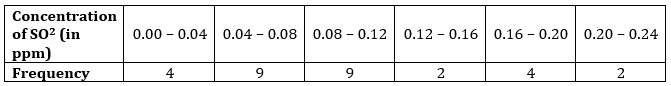Find the mean concentration of SO2 in the air.

Ans.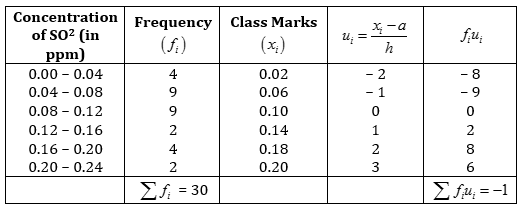From given data, Assume mean= 0.10, Width of the class= 0.04=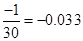(approx.)

Using formula, Mean= 0.10 + 0.04 (– 0.033) = 0.10 – 0.0013 = 0.0987 (approx.)

Hence mean concentration of SO2 in air is 0.0987 ppm.

NCERT Solutions for Class 10 Maths Exercise 14.1

###### 8. A class teacher has the following absentee record of 40 students of a class for the whole term. Find the mean number of days a student was absent.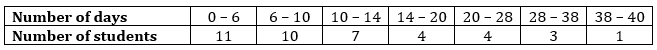Ans.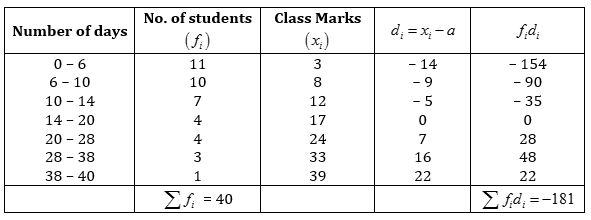From given data, Assume mean= 17=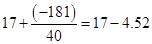= 12.48

Hence mean 12.48 number of days a student was absent.

NCERT Solutions for Class 10 Maths Exercise 14.1

###### 9. The following table gives the literacy rate (in percentage) of 35 cities. Find the mean literacy rate.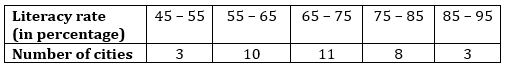Ans.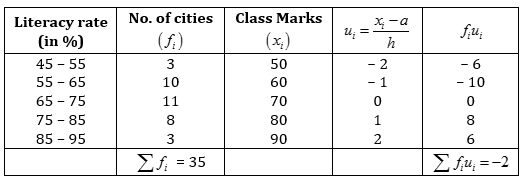From given data, Assume mean= 70, Width of the class= 10=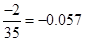Using formula, Mean= 70 + 10 (– 0.057) = 70 – 0.57 = 69.43

Hence mean literacy rate is 69.43%.

## NCERT Solutions for Class 10 Maths Exercise 14.1

NCERT Solutions Class 10 Maths PDF (Download) Free from myCBSEguide app and myCBSEguide website. Ncert solution class 10 Maths includes text book solutions from Mathematics Book. NCERT Solutions for CBSE Class 10 Maths have total 15 chapters. 10 Maths NCERT Solutions in PDF for free Download on our website. Ncert Maths class 10 solutions PDF and Maths ncert class 10 PDF solutions with latest modifications and as per the latest CBSE syllabus are only available in myCBSEguide.

## CBSE app for Class 10

To download NCERT Solutions for Class 10 Maths, Computer Science, Home Science,Hindi ,English, Social Science do check myCBSEguide app or website. myCBSEguide provides sample papers with solution, test papers for chapter-wise practice, NCERT solutions, NCERT Exemplar solutions, quick revision notes for ready reference, CBSE guess papers and CBSE important question papers. Sample Paper all are made available through the best app for CBSE### 5 thoughts on “NCERT Solutions for Class 10 Maths Exercise 14.1”

1. Nice

2. Good

3. in qus no. 5 no. of boxes kept varying inthe questionand answer

4. Thank you for your helping